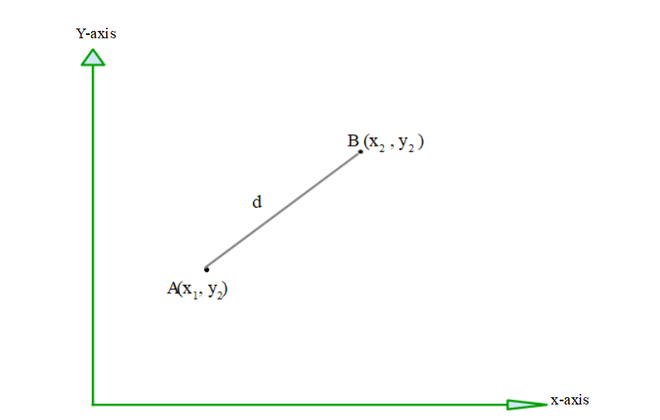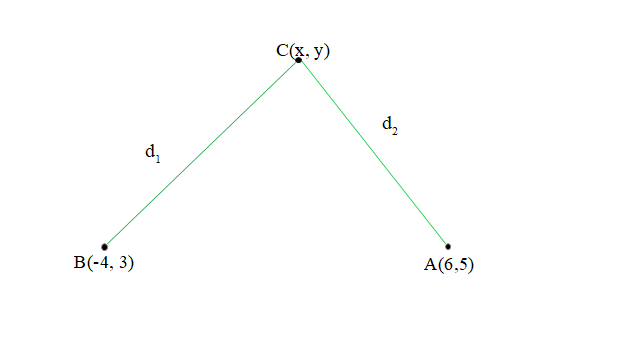GeeksforGeeks App
Open AppBrowser
Continue

# Find a relation between x and y such that the point (x, y) is equidistant from (6, 5) and (-4, 3)

Mathematics is a subject that is associated with numbers and calculations. And, according to the type of calculation mathematics is divided into different branches like algebra, geometry, arithmetic, etc. Geometry is the branch of mathematics that deals with shape and their properties. Geometry that deals with a point, lines, and planes in which coordinates are involved is called coordinate geometry.

### Coordinate geometry

Coordinate geometry is like a link between geometry and algebra through graphs. Using coordinate geometry distance between two points can be obtained, area of a triangle in a cartesian plane, etc. In coordinate geometry, the point is represented on the cartesian plane. A cartesian plane is a plane formed by two perpendicular lines that is the x-axis (horizontal axis) and the y-axis (vertical axis).

### Distance formula

This formula is used to calculate the distance between two points on a plane. In other words, it gives the length of the line segment which can be formed after joining two points. Let the two points be A and B, having coordinates to be (x1, y1) and (x2, y2) respectively. Then, the distance between two points is given as,Distance (d) =### Find a relation between x and y such that the point (x, y) is equidistant from (6, 5) and (-4, 3).

Solution:

Equidistant means having equal distance. (x, y) is equidistant from points (6, 5) and (-4, 3), which means a distance of the point (x, y) from both the point is equal. Let’s suppose A and B be the name of points (6, 5) and (-4, 3) respectively and C be the point having coordinated (x, y).According to the problem statement, d1 ( CB)  is equal to d2 (AC).

Use distance formula to find d1 and d2 values,

• d2 (Distance between (x, y) and (6, 5))=√(x2 – 12x + y2 – 10y + 61)

• d1 (Distance between (x, y) and (-4, 3))

==√(x2 + 8x + y2 – 6y + 25)

According to the question, d1 = d2

After substituting the values,

√(x2 – 12x + y2 – 10y + 61) = √(x2 + 8x + y2 – 6y + 25)

Squaring both sides,

(x2 – 12x + y2 – 10y + 61) = (x2 + 8x + y2 – 6y + 25)

x2 – x2 + y2 – y2 -10y + 6y – 12x – 8x = 25 – 61

-4y – 20x = – 36

4y + 20x = 36

y + 5x = 9

y = 9 – 5x

Hence, the relation between x and y such that the point (x, y) is equidistant from (6, 5) and (-4, 3) is y = 9 -5x

### Similar Problems

Question 1:  Find a relation between x and y such that the point (x, y) is equidistant from (0, 0) and (6, 6).

Solution:

(x, y) is equidistant from point (0, 0) and (6, 6), which means distance of point (x, y) from both the point is equal.

• d1(Distance between (x, y) and (0, 0))• d2(Distance between (x, y) and (6, 6))= √(x2 – 12x + y2 – 12y + 72)

According to the question, d1 = d2

After substituting the values:= √(x2 – 12x + y2 – 12y + 72)

Squaring both sides,

x2 + y2 = x2 – 12x + y2 – 12y + 72

x2 – x2 + y2 – y2 = -12x – 12y + 72

-72 = -12x – 12y

72 = 12x + 12y

6 = x + y

x = 6 – y

Hence, the relation between x and y such that the point (x, y) is equidistant from (0, 0) and (6, 6) is x = 6 – y

Question 2: Find a relation between x and y such that the point (x, y) is equidistant from (5, 0) and (0, 5).

Solution:

(x, y) is equidistant from point (5,0) and (0,5), which means distance of point (x,y) from both the point is equal.

• d1 (Distance between (x, y) and (5, 0))= √{x2 – 10x +25 + y2}

• d2 (Distance between (x, y) and (0, 5))=√(x2 + y2 – 10y + 25)

According to the question, d1 = d2

After substituting the values:

√(x2 – 10x + 25 + y2) = √(x2 + y2 – 10y + 25)

Squaring both sides,

x2 – 10x + 25 + y2 = x2 + y2 – 10y + 25

x2 – x2 + y2 – y2 = -10y +10x + 25 – 25

10y = 10x

y = x

Hence, the relation between x and y such that the point (x, y) is equidistant from (0, 5) and (5, 0) is x = y

My Personal Notes arrow_drop_up
Related Tutorials